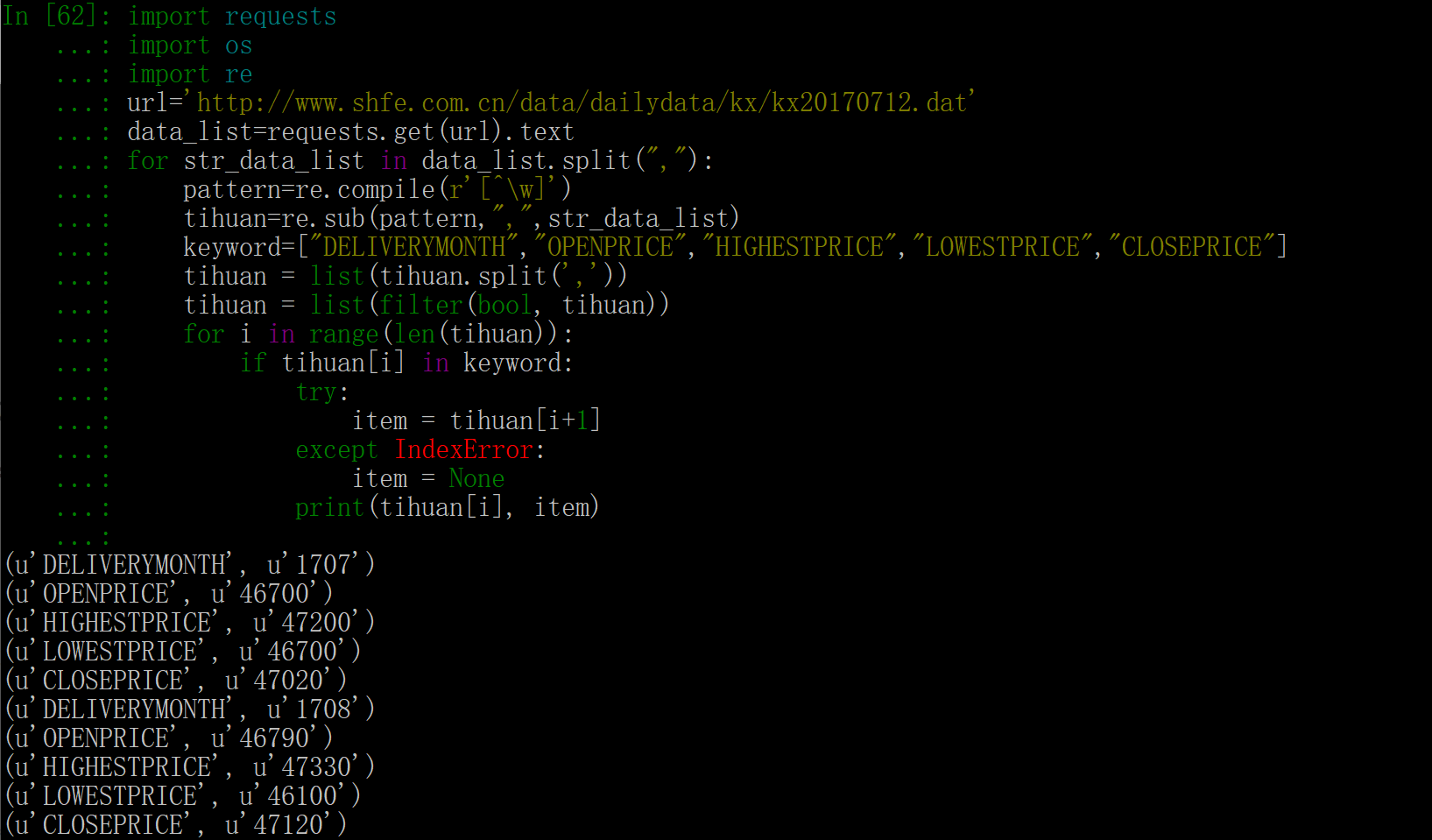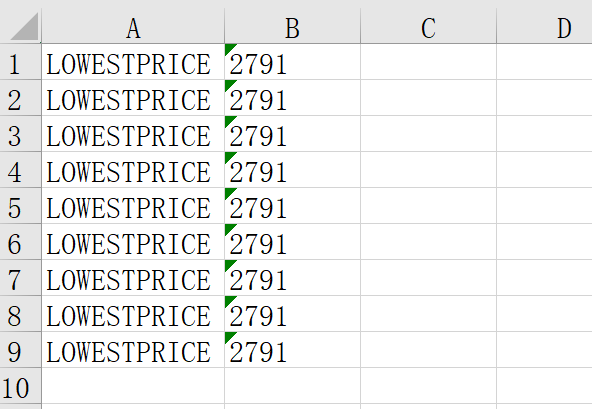python获取数据后导入excel问题？import requests
import os
import re
from openpyxl.workbook import Workbook

url='http://www.shfe.com.cn/data/dailydata/kx/kx20170714.dat'
data_list=requests.get(url).text
for str_data_list in data_list.split(","):
pattern=re.compile(r'[^\w]')
tihuan=re.sub(pattern,",",str_data_list)
keyword=["DELIVERYMONTH","OPENPRICE","HIGHESTPRICE","LOWESTPRICE","CLOSEPRICE"]
tihuan = list(tihuan.split(','))
tihuan = list(filter(bool, tihuan))
for i in range(len(tihuan)):
if tihuan[i] in keyword:
try:
item = tihuan[i+1]
except IndexError:
item = None
wb=Workbook()
ws=wb.worksheets
arr1=[]
arr2=[]
for row in range(1,10):
a1=ws.cell(row=row,column=1,value="{0}".format(tihuan[i]))
a2=ws.cell(row=row,column=2,value="{0}".format(item))
if a1:
arr1.append(a1)
if a2:
arr2.append(a2)
wb.save('test.xlsx')1个回答

for row in range(1,10):
a1=ws.cell(row=row,column=1,value="{0}".format(tihuan[i]))
a2=ws.cell(row=row,column=2,value="{0}".format(item))